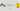Sapnesh Naik
Software Developer, Technical Writer
sapnesh@kerneldev.com
Blog Post

# getopt - Command Line Arguments in Python [Part 2]

September 5, 2018In the last article, we learned how to handle command line arguments in Python using sys.argv. We also noticed that it is not the ideal solution if your use case is a bit more complex. Now let’s see getopt,- a python module which builds on `sys.argv`. `getopt` provides us with features that make it easier to process command line arguments in Python.

We will be writing a simple python script to add or subtract two numbers and see how `getopt` is better than just using `sys.argv`.

### Using Getopt:

• `getopt` is a module that comes bundled with any standard python installation and therefore you need not install it explicitly.
• A major advantage of `getopt` over just using `sys.argv` is `getopt` supports switch style options ( Ex,`-s` or `--sum`).
• Hence `getopt` supported options are position-independent. The example `\$ ls -li` works the same as `\$ ls -il`
• The options are of two types:

• Options that need a value to be passed with them. These are defined by the option name suffixed with `=` (Ex, `num1=`)
• Options that behave as a flag and do not need a value. These are defined by passing the option name without the suffix `=` (Ex, `--subtract`)
• The options can have two variations:

• `shortopts` are one letter options, denoted by prefixing a single `-` to an option name (Ex, `\$ ls -l`)
• `longopts` are a more descriptive representation of an option, denoted by prefixing two `–` to an option name (Ex, `\$ ls --long-list`)
• As we saw in the `sys.argv` tutorial the options in `getopt`are always parsed as`string`. So be careful if you want your input to have any other type. You may need to cast or convert the elements according to your requirements.
``````#!/usr/bin/python
# -*- coding: utf-8 -*-

import getopt
import sys

#print script usage
def usage():
print("Addition: python get_opt.py -a <number1> -b <number2>")
print("Subtraction: python get_opt.py -a <number1> -b <number2> --subtract")
print('Example: python get_opt.py -a 10 -b 3\n')

#return sum of two numbers
return a + b

#return difference of two numbers
def subtract(a, b):
return a - b

def main():
try:
(opts, args) = getopt.getopt(sys.argv[1:], 'ha:b:s', ['help','num1=', 'num2=', 'subtract'])
#raised whenever an option (which requires a value) is passed without a value.
except getopt.GetoptError as err:
print(err)
sys.exit(2)

num1 = num2 = None
sub = False

#check if any options were passed
if len(opts) != 0:
# loop over opts: Ex, [(a,10), (b,20)] => <list>
for (o, a) in opts:
if o in ('-h', '--help'):
usage()
sys.exit()
elif o in ('-a', '--num1'):
num1 = int(a)
elif o in ('-b', '--num2'):
num2 = int(a)
elif o in '--subtract':
sub = True
else:
#none of the acceptable options were passed
usage()
sys.exit(2)
else:
#no options were passed
usage()
sys.exit(2)

#check if num1 and num2 not None
if num1 and num2:
if sub:
print ('\n Difference in two numbers is :', subtract(num1, num2), '\n')
else:
print ('\n Sum of two numbers is :', add(num1, num2), '\n')
else:
usage()
sys.exit(2)

if __name__ == '__main__':
main()``````

### Explaining the Code:

• Our code prints the sum (addition) of the two numbers (`-a` and `-b`) but it can also print the difference between those numbers (subtraction) if you pass the flag `--subtract` or `-s`

``(opts, args) = getopt.getopt(sys.argv[1:], 'ha:b:s', ['help','num1=', 'num2=', 'subtract'])``
• getopt module provides a `getopt(args, shortopts, longopts=[])` function which we can use to define our options.
• sys.argv holds the unformatted list of all the arguments passed to a python script. Read more about it here.
• We’re using two variables (`opts`, `args`) because getopt.getopt function returns two elements. One containing a `<list>` of options and another has a `<list>` of arguments that are not specified in our getopt initialization.
• You can specify `shortopts` as a colon(:) separated single letter characters.
• You can specify `longopts` as a comma-separated list of words with the suffix `=`
• `longopts` without the suffix `=` are considered as a flag and they should be passed without any value.
• Now, to use the options passed to our program we can just iterate over the `opts` variable like any other list.

``for (o, a) in opts:``

Here `o` will hold our option name and `a` will have any value assigned to the option. Also notice that as `--subtract` is being used as a `flag` it will not have any value. I am using this flag to decide whether to print the sum of the inputs or the difference in them.

### So when Should I Use or Not Use Getopt?

• `getopt` provides an easy way to add switch-like, position-independent options in your script. You can quickly get up and running with it.
• But `getopts` falls short if your requirements are a bit more specific, such as you need the arguments to be of certain data type or you need to make certain options mandatory. In such a case, you should take a look at `argparse`, Python’s recommended module for command-line argument parsing.